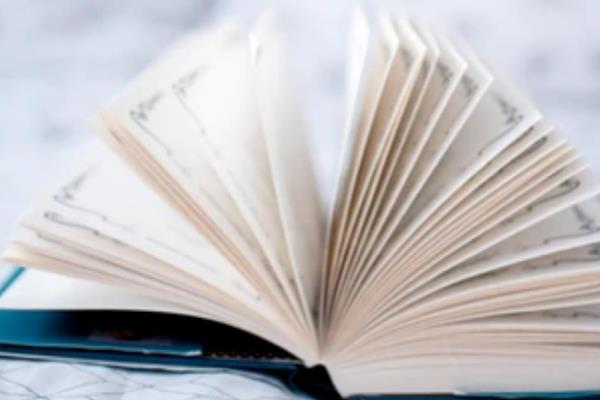# 小学一年级上册数学练习题【5篇】1.小学一年级上册数学练习题

一、填一填

1、18前面第3个数是(   )，13后面第5个数是(   )。

2、(   )比19多1，(   )比19少1。

3、一个两位数十位是1、个位上的数比十位多6，这个数前面一个数是(   )

4、今年爸爸比妈妈大3岁，3年后妈妈比爸爸小(   )岁。

5、小明和小红有同样多的苹果，小明吃了2个，小红吃了3个，(   )剩下的多。

二、画一画

1、△△□△△□△△□____、____、____

○△○○△○○○△____、____、____、____

2、画△，比○多1个○○○○○○○○○○

________________________

三、解决问题

1、一包糖，小东吃了4粒，小华吃了6粒，还剩下10粒。这包糖原来有几粒？

2、找规律填数。

(1)2、4、6、8、10、(   )

(2)20、15、10、(   )

3、14个小朋友玩捉迷藏，已经捉住了4个小朋友，还藏着(   )个小朋友。

4、小红前面5人，后面7人，一共有(   )人。

5、□比○多2个，□□□□□□，○有(   )个。

2.小学一年级上册数学练习题

一、在○里填上“＞”、“＜”或“=”。

8+5○14　　14-4○10　　7+8○8+7

5+9○6+7　　9○4+6　　10○10-2

二、在○里填上“+”或“-”。

3○6=9　　8○5=3　　12○2=10

10○4=14　　18○10=8　　7○7=0

10○9=19　　6○6=12　　5○4=9

7○2=5　　7○9=16　　9○1=8

三、看谁算得又对又快。

9+2=　　8+3=　　7+4=

6+7=　　7+5=　　4+6=

9+8=　　6+5=　　5+4=

10－3=　　9－8=　　7－6=

8－5=　　9－9=　　6+6=

10+5=　　6+8=　　15－10=

13+2=　　4+10=　　17－7=

4+9=　　10－9=　　9－7=

3+1+4=　　9－7－1=　　7+0+1=

2+7－3=　　10－6+5=　　9+9－10=

8－4+3=　　8－6+7=　　10－9+6=

5+8－3=　　6+6－2=　　7－7+5=

3.小学一年级上册数学练习题

一、口算。

5+2=　　9+3=　　6+5=　　8+4=　　9－5=

10－6=　　3+6=　　6+4=　　18－8=　　7－7=

7+8=　　9+6=　　6+7=　　10－7=　　3+5=

7+4=　　8+9=　　7+7=　　12－2=　　9－6=

2+9=　　5+8=　　7+9=　　9+4=　　9+5=

1+9=　　12－10=　　6+10=　　6+8=　　6+9=

二、按顺序填空。

（1）__________6__________9_____。

（2）14__________17_______________。

（3）19_______________15__________。

三、填空。

（1）9添上1是（），19添上1是（）。

（2）16里面有（）个十和（）个一。

（3）1个十是（）个一。

（4）大于11又小于13的数是（）。

（5）一个两位数，从右边起第一位是（）位，第二位是（）位。

4.小学一年级上册数学练习题

1、有4只小兔，小猴的只数是小兔的3倍，现在每2只小猴分成一组去抬东西，可以分成几组？

2、马场上有29匹马，又来了15匹，现在马场上有多少匹？

3、小苹种7盆红花，又种了同样多的黄花，两种花共多少盆？

4、学校买回白粉笔37盒，彩色粉笔8盒，彩色粉笔比白粉笔少多少盒？

5、每条船上坐8人，4条船能坐多少人？

6、小林吃了8块饼干后，小林现在有4块饼干，小林原来有多少块饼干？

7、刚刚有9本书，爸爸又给他买了5本，小明借去2本，刚刚还有几本书？

8、云云画了6面旗，红红画了5面，他们一共画了多少面？

9、妮妮有16本作业本，用了8本，还剩多少本？

10、学校买回白粉笔37盒，彩色粉笔8盒，彩色粉笔比白粉笔少多少盒？

11、明明要剪12个五角星，剪好了7个，还要剪几个？

12、学校买回白粉笔37盒，彩色粉笔8盒，买回粉笔共多少盒？

13、13只鸡排成一队，其中有只大公鸡，从前面数，它站在第8，它的后面有几只鸡？

14、我有50元，要买一件29元的衣服和一副18元的眼镜，还剩多少元？（两种方法）

15、王心看故事书，看了12页，还剩7页，这本书有多少页？

5.小学一年级上册数学练习题

（1）吃饭时，我们通常用（）手端碗，（）手拿筷子。

（2）写字时，我们通常用（）手拿笔。

（3）走路时，我们要靠（）侧行走。

（4）上课发言应举（）手，走路时应先迈（）脚。

（5）10前面有（）个数，6再往后数的三个数是（）、（）、（），6往前数两个数是（）、（）。

（6）1个十和5个一合起来是（）。

（7）2个十组成的数是（）。

（8）比14多1的数是（），比14少1的数是（）。

（9）与17相邻的两个数分别是（）和（）。

（10）16里面有（）个十和（）个一。

（11）1个十和5个一合起来是（）；1个十和（）个一合起来是（）。

（12）和18相邻的两个数是（）和（）。

（13）16比10多（）。8比14少（）。

（14）12前面一个数是（），后面一个数是（）。

（15）14里面有（）个一和（）个十。

（16）9个一和1个十合起来是（）。

（17）10前面一个数是（），后面一个数是（）。

（18）20里面有（）个十。

（19）我比17小，比15大，我是（）。

（20）我在14和12之间，我是（）。

（21）13的个位上是（），十位上是（）。